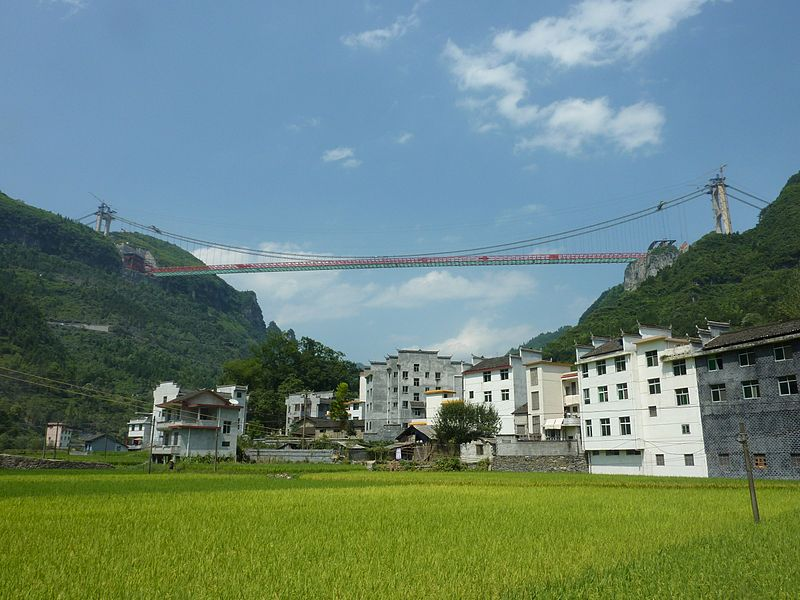Filippo Gazzola
INTERESSI SCIENTIFICI
SCIENTIFIC INTERESTS

Mathematical models for suspension bridges: fourth order PDE models, fourth order ODE models, Hamiltonian systems, design for stiffening trusses.

Critical point theory: semilinear and quasilinear elliptic equations at critical growth, quasilinear elliptic problems via nonsmooth critical point theory, periodic motions in Hamiltonian systems consisting in lattices of particles, N-body problem.
Higher order partial differential equations: elliptic equations at critical growth, Steklov boundary conditions.
Ground states for quasilinear elliptic problems: existence results and asymptotic behavior for the p-Laplacian, existence results for the prescribed mean curvature operator.
Calculus of variations: shape optimization, approximation of the infima of (nonconvex) scalar functionals and of the minimizing functions, symmetry problems.
Sobolev, Hardy, Rellich inequalities: inequalities with remainder terms.
Navier-Stokes equations: modified NS equations, NS equations with a pressure-dependent viscosity.
Characteristic hyperbolic problems: inflow-outflow problems for Euler equations of compressible fluids.
Dynamical systems: attractors in weak topologies of Banach spaces.AIZHAI SUSPENSION BRIDGE (China, 2012)AIZHAI SUSPENSION BRIDGE (China, 2012)